Next: Tree Construction Algorithm Up: Using Traveling Salesman Problem Previous: Finding a circular order

# Error Bound

Before we present the tree construction algorithm, we want to determine how big the errors of the distance measurement can be in order to get a correct circular tour.

Definition 3.1   Let L be a set of n leaves of a tree T. The distance between two leaves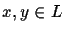is d(x, y). It is the unique path length from leaf x to leaf y. We assume that distances are symmetric, hence d(x, y) = d(y, x).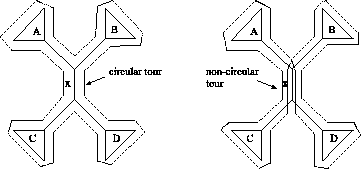Given is a tree T (see Figure 3). We want to find the maximum error of the distance measurement, such that the circular order C' derived from an exact TSP algorithm is incorrect, and therefore at least one edge x (the shortest edge) is traversed more than twice. A correct circular order C will pass edge x exactly twice. Now assume that our distances have some errors.t we get an incorrect order C' (right side of Figure 3). In both cases, subtrees A, B, C and D are all traversed in the same way, so we can represent them with any leaf in the subtree. If we have a wrong circular order C', the following inequality must be satisfied:
d(a,b)+ d(b, c)+ d(c, d)+d(d, a) > d(a, d)+ d(d, b) + d(b, c)+d(c, a)
We now include the errorsin the inequality and simplify the above inequality: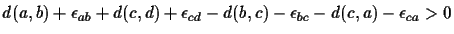where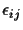is the error in the distance measurement d(i,j). But when our distance matrix would be additive, without any error, then the following equation holds (see Figure 4):
d(a, b) + d(c, d) - d(b, c) - d(c, a) = -2x
If we subtract the second from the first equation, we get: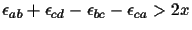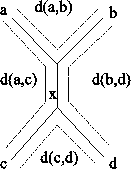The conclusion is: we only get a wrong circular order if each of the four distances involved has an error of at least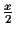(the length of the shortest edge).
Note that this is the error if we simply take any random circular order C. But we do not take a random circular order, our circular order is the solution of a TSP algorithm. Hence to get a wrong tour, all other circular tours that involve the same distances to cross edge x must also be larger than the wrong order C'. Otherwise, if just one of the other correct circular orders Ci would yield a shorter tour than C', then the TSP algorithm must return the correct tour Ci.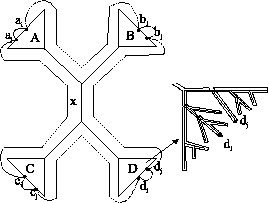First we have to determine the number of circular orders we have to consider. We want to find the error with respect to the shortest edge x. So we have to figure out how many different circular orders there are for traversing x. In Figure 5 this situation is depicted: for the subtree A there are |A| possible ways to start and |A| end the cycle in the subtree. The same accounts for subtrees B, C and D. So there are in total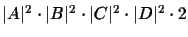circular orders that have to be greater than the wrong order C' (the 2 comes from interchanging the subtrees themselves). Let p be the probability that a circular order C is wrong in terms of the shortest edge x. Then the probability that the TSP order is wrong is: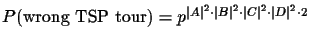For illustration we give a small example: let the probability p=0.99, that means that 9 out of 10 circular orders are wrong. Let us take a tree with 8 leaves, s.t. |A|=|B|=|C|=|D|=2, so there are 512 circular orders that must be greater than the wrong order C'. The the probability to get a wrong TSP order is then: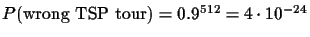Next: Tree Construction Algorithm Up: Using Traveling Salesman Problem Previous: Finding a circular order
Chantal Korostensky
1999-07-14